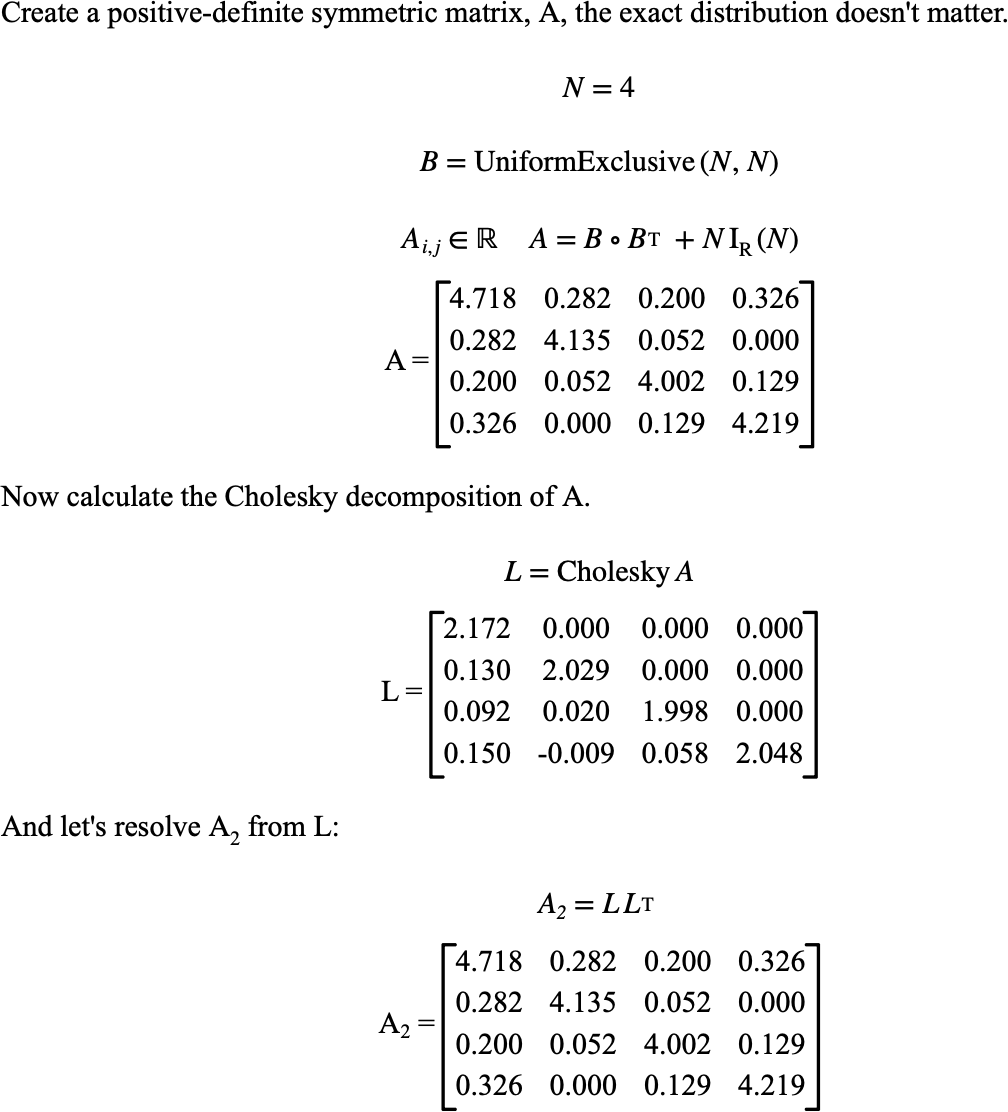# $$\text{Cholesky}$$¶

You can use the $$\text{Cholesky}$$ function to perform a Cholesky decomposition of a real or complex matrix, creating $$L \times L^T$$ for real matrices and $$L \times L^H$$ for complex matrices.

You can use the \cholesky backslash command to insert this function.

The following variants of this function are available:

• $$\text{real matrix } \text{Cholesky} \left ( \text{<boolean matrix>} \right )$$

• $$\text{real matrix } \text{Cholesky} \left ( \text{<integer matrix>} \right )$$

• $$\text{real matrix } \text{Cholesky} \left ( \text{<real matrix>} \right )$$

• $$\text{complex matrix } \text{Cholesky} \left ( \text{<complex matrix>} \right )$$

The $$\text{Cholesky}$$ function returns the lower triangular matrix. You can use use the transpose or Hermitian operator to calculate the second matrix term.

Note that this function is only defined for symmetric positive-definite matrices (or Hermitian positive-definite matrices). The $$\text{Cholesky}$$ function will trigger a run-time error or return an empty matrix if the input matrix does not meet this constraint.

The function is implemented internally for real and complex matrices although you can supply boolean and integer matrices which will be converted to real matrices prior to performing the decomposition operation.

Figure 120 shows the basic use of the $$\text{Cholesky}$$ function.Figure 120 Example Use Of The Cholesky Function